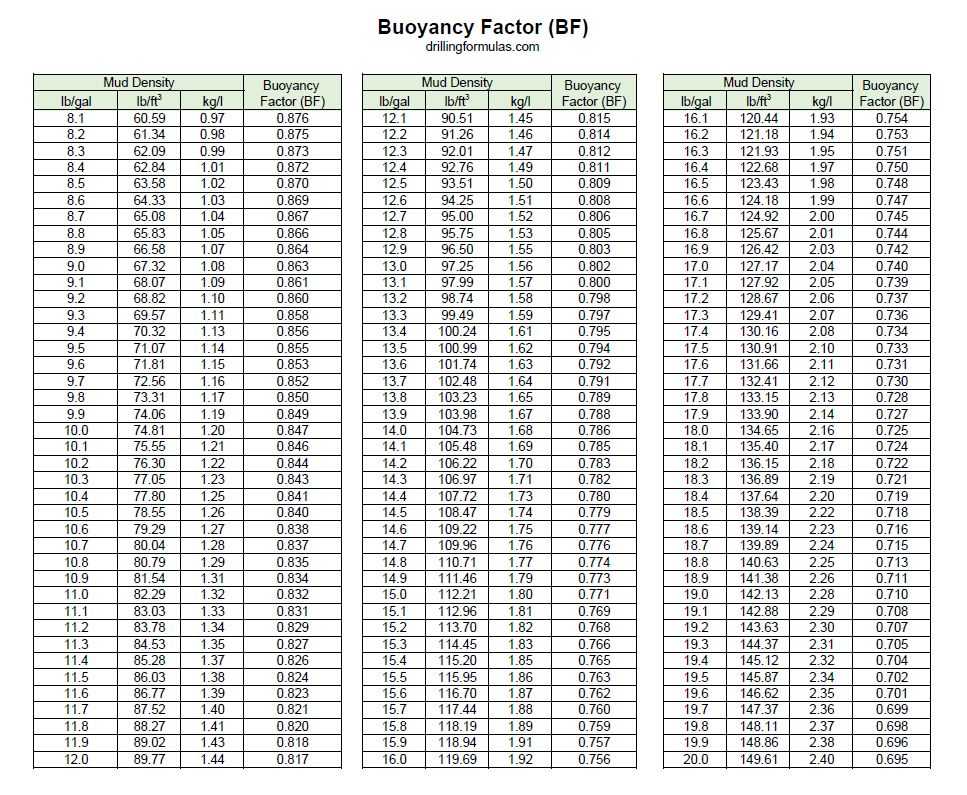# Buoyancy Factor Calculation

Buoyancy Factor is the factor that is used to compensate loss of weight due to immersion in drilling fluid.

Before explaining any further, we will explain you about the basic concept of Buoyancy. Buoyancy is the upward force that keeps things afloat. The net upward buoyancy force equals to the amount of the weight of fluid displaced by the body volume. This force will make objects lighter when it immerses in fluid. For example, we feel ourselves lighter when we are in swimming pool because this is the effect of buoyancy.In drilling operation, we need to know how much weight of string of drill pipe, completion string, etc in drilling fluid. Therefore,  Buoyancy Factor is value that we need to know and be able to calculate this value. Please follow the formulas below to calculate Buoyancy Factor in different mud weight units, ppg and lb/ft3.

## Buoyancy Factor using mud weight in ppg

Buoyancy Factor (BF) = (65.5 – mud weight in ppg) ÷65.5

Note: 65.5 ppg is density of steel.

Example: Determine the buoyancy factor for a 13.0 ppg fluid:
BF = (65.5 – 13.0) ÷ 65.5
BF = 0.8015

## Buoyancy Factor using mud weight in  lb/ft3

Buoyancy Factor (BF) = (489 – mud weight in lb/ft3) ÷489

Note: 489 lb/ft3 is density of steel.

Example: Determine the buoyancy factor for a 100 lb/ft3 fluid:
BF = (489 – 100) ÷489
BF = 0.7955

## Buoyancy Factor using mud weight in kg/l

Buoyancy Factor (BF) = (7.85 – mud weight in kg/l) ÷7.85

Note: 7.85 kg/l is density of steel.

Example: Determine the buoyancy factor for a 1.1 kg/l fluid:
BF = (7.85 – 1.1) ÷7.85
BF = 0.860

## Buoyancy Factor Table

This table demonstrates buoyancy factor at different mud densityBuoyancy Factor Table

## How to use the Buoyancy Factor to determine buoyed weight

In order to figure out the actual weight of drilling string in fluid, the air weight of drilling string times the buoyancy factor equal to actual weight in mud, called buoyed weight.

Buoyed weight of drill string = String weight in the air × Buoyancy Factor

Example: Determine the string weight in 13.0 ppg mud. Air weight of string is 350 klb.

The buoyancy factor for a 13.0 ppg fluid:
BF = (65.5 – 13.0) ÷ 65.5
BF = 0.8015

Buoyed weight of drill string = String weight in the air × Buoyancy Factor

The buoyed weight of drill string in 13.0 ppg mud = 350 x 0.8015 = 280.5 Klb.Ref books:

Lapeyrouse, N.J., 2002. Formulas and calculations for drilling, production and workover, Boston: Gulf Professional publishing.

Bourgoyne, A.J.T., Chenevert , M.E. & Millheim, K.K., 1986. SPE Textbook Series, Volume 2: Applied Drilling Engineering, Society of Petroleum Engineers.

Mitchell, R.F., Miska, S. & Aadny, B.S., 2011. Fundamentals of drilling engineering, Richardson, TX: Society of Petroleum Engineers.

Share the joyWorking in the oil field and loving to share knowledge.

### 20 Responses to Buoyancy Factor Calculation

1.Drilling Fluid says:

Hi, during drilling process we need to know how much weight of string of drill pipe, completion string, etc in drilling fluid. Buoyancy Factor is value that we need to know and be able to calculate this value.

Regards

•DrillingFormulas.Com says:

During drilling or running completion, you can see the actual weight from your weight indicator. That number is already accounted for Buoyancy factor already.

2.kingsley says:

please i need write up on evaluation of drilling bit performance

•DrillingFormulas.Com says:

Could you give me more details?

3.URL says:

It is hard to locate knowledgeable folks on this subject, but you sound like you know what you are talking about! Thanks 537099

4.sam soh says:

what happens if my well is completed with a 12.1 ppg completion fluid, i then go on to displace tubing fluid to 10.3 ppg. how do i find out the wet weight of the tubing string ? displacing of tubing fluid occurs before packer has set.

•DrillingFormulas.Com says:

Your weight showing in the weight indicator will slightly increase because of reduction of density. In order to get the weight in the completion fluid, you determine the new buoyancy factor using 10.3 ppg and multiply by string weight.

Regards,
Shyne.

5.Rinat says:

Is there a difference between weight of the drill string and weight of the drill string in air? Thank you!

•DrillingFormulas.Com says:

Rinat,

Yes. Buoyed weight of drill string = String weight in the air x Buoyancy Factor

6.Leon says:

How do you calculate the bouyoncy force on the cutting of weight 8.33 ppg and the volume of mud is 3 in3 with a weight of 11ppg

•DrillingFormulas.Com says:

Leon,

The cutting weight in your example is too low. It will float in the drilling mud.

Regards,
Shyne

7.Luciano says:

What is the 65,5 on the formula for bouyancy fator?

•DrillingFormulas.Com says:

65.5 ppg is metal density.

8.Mero says:

How the buyouancy factor affect the string Wt if the string is stinged into packer?

•DrillingFormulas.Com says:

Mero,

The buoyancy factor still has the same effect since the string is submersed into fluid.

9.ddan says:

There are US and UK types of pounds per gallon density. Which one can be used in buoyancy factor calculation?
Thanks

•DrillingFormulas.Com says:

US gallon is used for this calculation.

10.Sai says:

why is the buoyant weight of drill string important?
Does it play a role in the load provided/rotary motion provided.

11.guest says:

Very Important Note. This formula for unplugged pipe at the bottom.

12.Hassan says:

Thanks for the information, good job

This site uses Akismet to reduce spam. Learn how your comment data is processed.